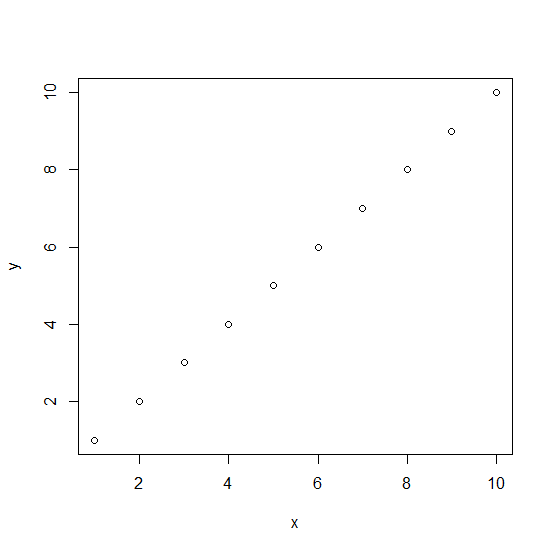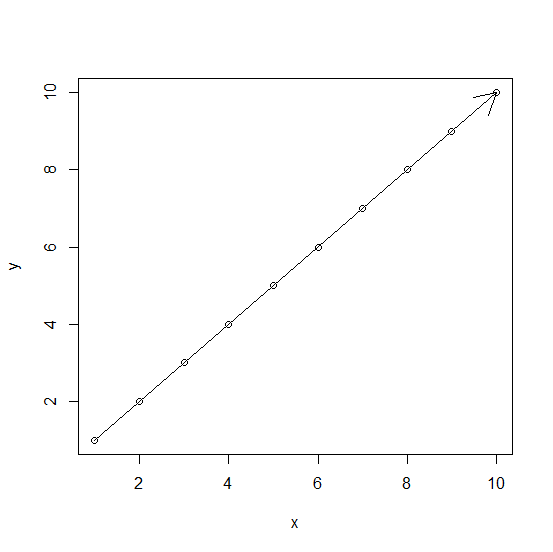# How to create an arrow in base R?

R ProgrammingServer Side ProgrammingProgramming

To create an arrow R, we can use plot function and arrows function. We just need to understand all the coordinate values that should be passed inside the arrows function. For example, if we have two vectors that contains values from 1 to 10 then the arrow can be created by using arrows function as arrows(1,1,10,10).

## Example

Live Demo

> x<-1:10
> y<-1:10
> plot(x,y)

## Output## Example

> arrows(1,1,10,10)

## Output